### 15.4.2.4 The Frobenius Theorem

The Lie bracket is the only tool needed to determine whether a system is completely integrable (holonomic) or nonholonomic (not integrable). Suppose that a system of the form (15.53) is given. Using the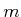system vector fields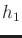,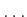,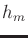there are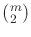Lie brackets of the form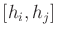for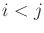that can be formed. A distribution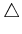is called involutive  if for each of these brackets there existcoefficients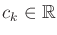such that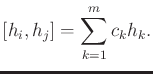(15.86)

In other words, every Lie bracket can be expressed as a linear combination of the system vector fields, and therefore it already belongs to. The Lie brackets are unable to escapeand generate new directions of motion. We did not need to consider all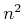possible Lie brackets of the system vector fields because it turns out that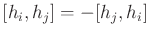and consequently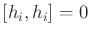. Therefore, the definition of involutive is not altered by looking only at thepairs.

If the system is smooth and the distribution is nonsingular, then the Frobenius theorem immediately characterizes integrability:

A system is completely integrable if and only if it is involutive.
Proofs of the Frobenius theorem appear in numerous differential geometry and control theory books [133,156,478,846]. There also exist versions that do not require the distribution to be nonsingular.

Determining integrability involves performing Lie brackets and determining whether (15.86) is satisfied. The search for the coefficients can luckily be avoided by using linear algebra tests for linear independence. The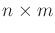matrix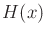, which was defined in (15.56), can be augmented into an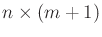matrix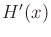by addingas a new column. If the rank ofis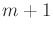for any pair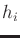and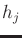, then it is immediately known that the system is nonholonomic. If the rank ofisfor all Lie brackets, then the system is completely integrable. Driftless linear systems, which are expressed as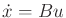for a fixed matrix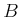, are completely integrable because all Lie brackets are zero.

Example 15..11 (The Differential Drive Is Nonholonomic)   For the differential drive model in (15.54), the Lie bracket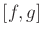was determined in Example 15.9 to be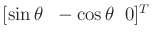. The matrix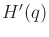, in which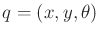, is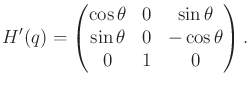(15.87)

The rank ofis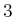for all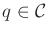(the determinant ofis). Therefore, by the Frobenius theorem, the system is nonholonomic.Example 15..12 (The Nonholonomic Integrator Is Nonholonomic)   We would hope that the nonholonomic integrator is nonholonomic. In Example 15.10, the Lie bracket was determined to be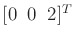. The matrixis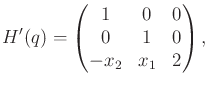(15.88)

which clearly has full rank for all.Example 15..13 (Trapped on a Sphere)   Suppose that the following system is given: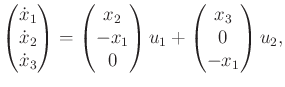(15.89)

for which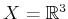and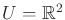. Since the vector fields are linear, the Jacobians are constant (as in Example 15.10):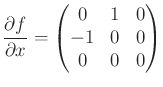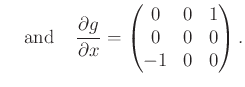(15.90)

Using (15.80),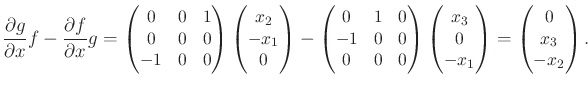(15.91)

This yields the matrix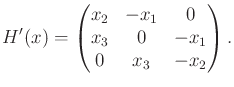(15.92)

The determinant is zero for all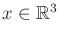, which means thatis never linearly independent of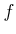and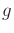. Therefore, the system is completely integrable.15.10

The system can actually be constructed by differentiating the equation of a sphere. Let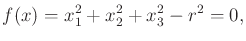(15.93)

and differentiate with respect to time to obtain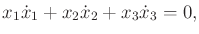(15.94)

which is a Pfaffian constraint. A parametric representation of the set of vectors that satisfy (15.94) is given by (15.89). For each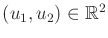, (15.89) yields a vector that satisfies (15.94). Thus, this was an example of being trapped on a sphere, which we would expect to be completely integrable. It was difficult, however, to suspect this using only (15.89).Steven M LaValle 2020-08-14